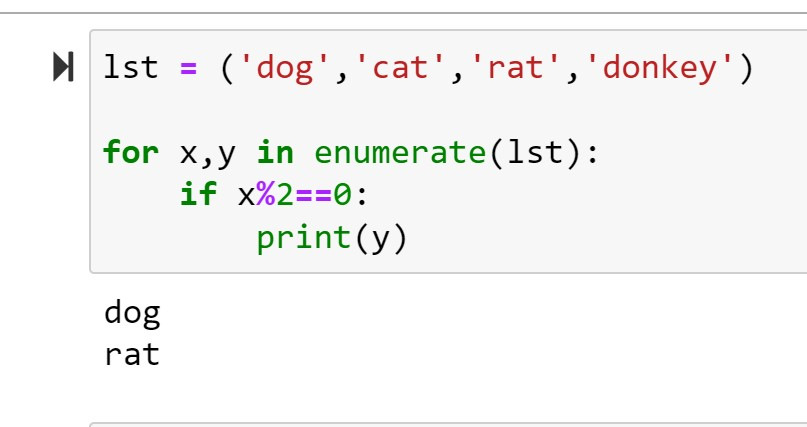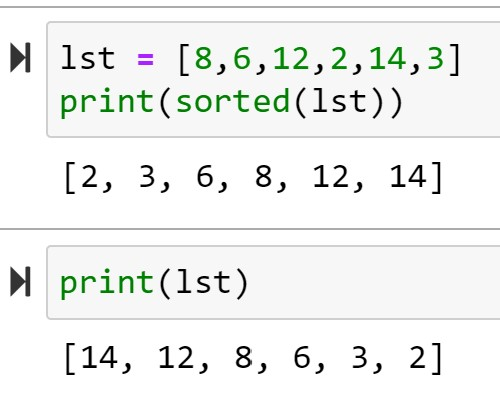# Python: Enumerate() and Sort

## Enumerate

Enumerate is a useful function in Python as it returns both the indexes and values of Lists.

Let’s look at the code:

Running the list through a For loop, we assign the index to x and the value to yLet’s say we only wanted to print every other value in a list

To do that, I could run a simple nested an IF statement inside my loop stating if the index an even number, then print the value. (x%2==0 is an easy trick for finding even or odd values. % means modulus – it returns the remainder of a division problem. Any even number divided by 2 will not have a remainder – so the modulus will be 0)Try your code in our online Python console:## Sort

Python lists come with a two built in sort functions.

### sorted()

The first: sorted() – sorts the items in a list, but does not alter the list.

As you can see, sorted(lst) returns a sorted list, but calling lst again shows the list is still in its original order.To sort the items in descending order use the reverse=True command:### list.sort()

list.sort() is a method – meaning it is a function found with the class List.

list.sort() sorts items in a list and alters position of the items in the actual list. So the next time you run the list, the items are sorted in the list.

Also note that reverse = True works in the list.sort() method as well.Try your code in our online Python console:If you enjoyed this lesson, click LIKE below, or even better, leave me a COMMENT.

Last Lesson: Functions

Next Lesson: Error Handling

Back to Python Course: Course

## One thought on “Python: Enumerate() and Sort”

1. Pingback: Python Functions – Analytics4All